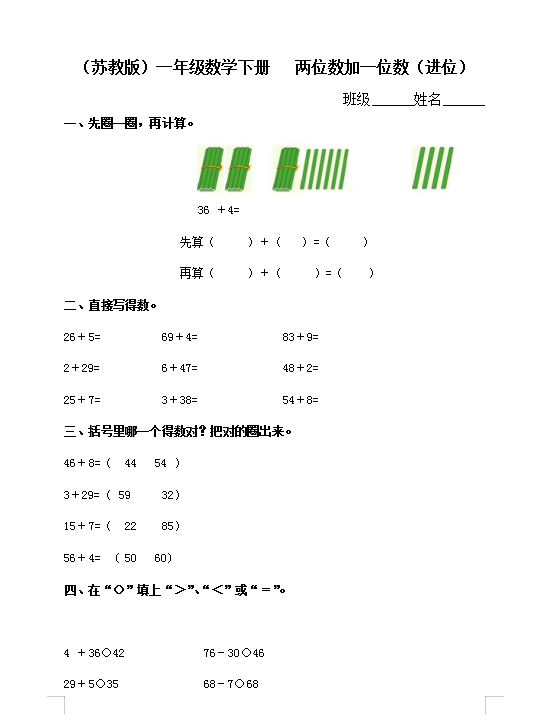（苏教版）小学一年级数学下册 两位数加一位数（进位）及答案（4页DOC文档）资料下载

（苏教版）小学一年级数学下册   两位数加一位数（进位）

26＋5=          69＋4=              83＋9=

2＋29=          6＋47=              48＋2=

25＋7=          3＋38=              54＋8=